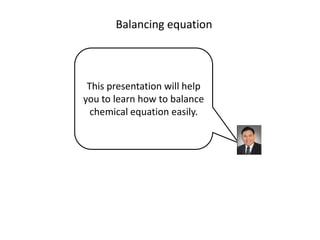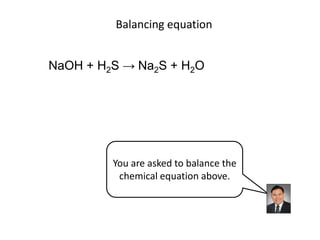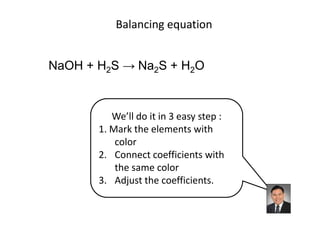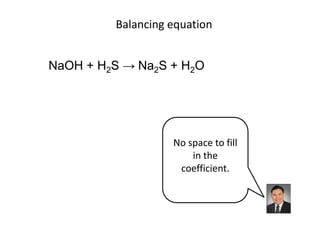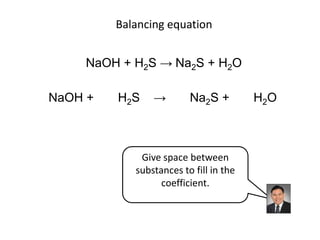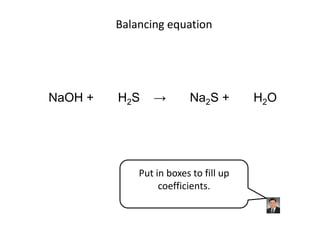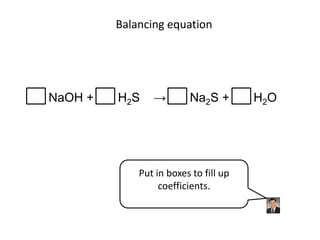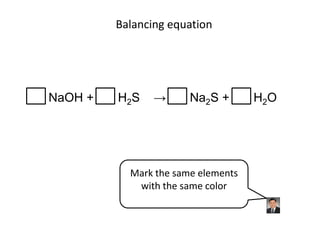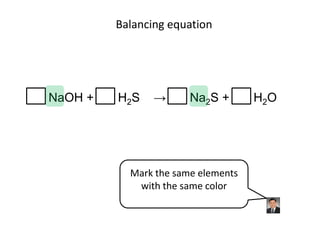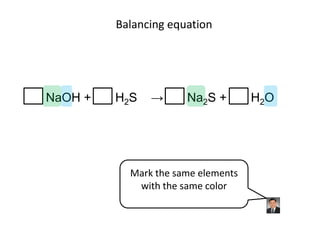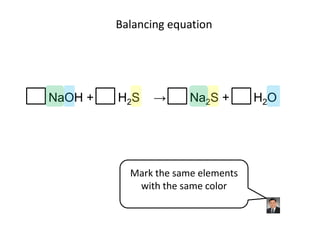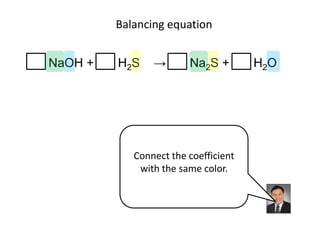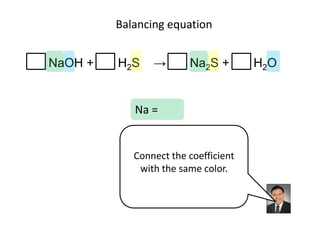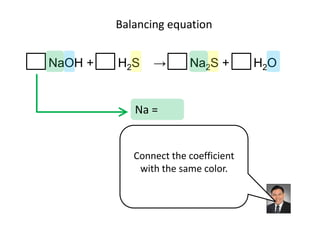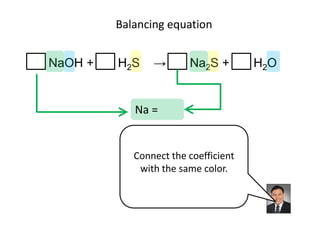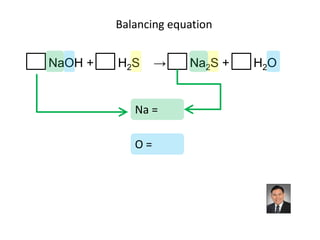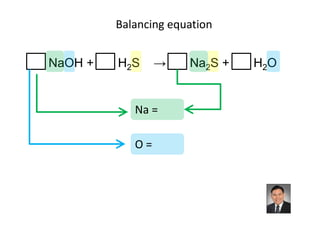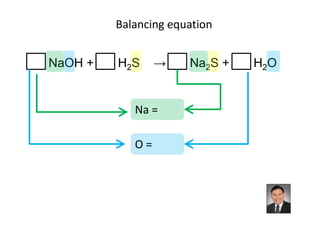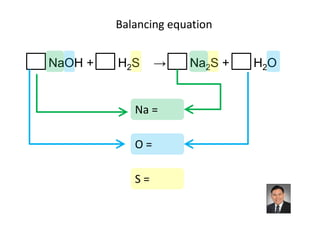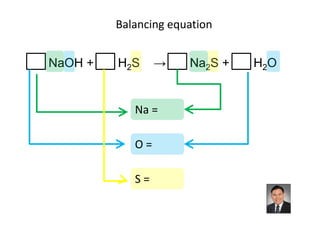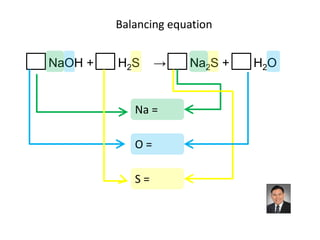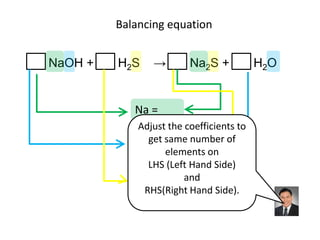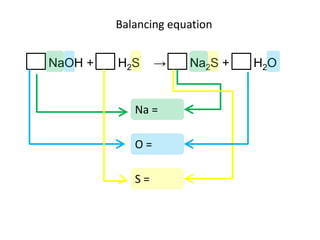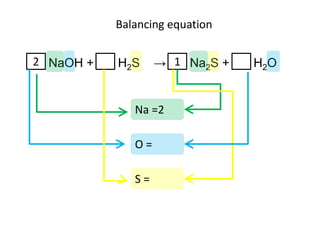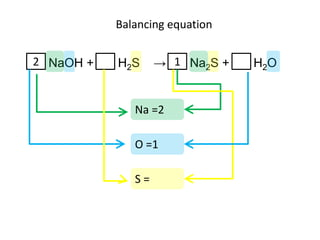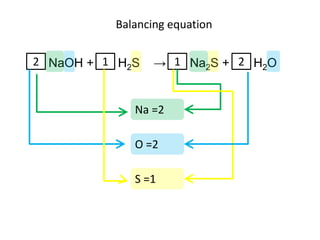1 of 27

### Balance Equation v004

1. Balancing equation This presentation will help you to learn how to balance chemical equation easily.
2. Balancing equation NaOH + H2S → Na2S + H2O You are asked to balance the chemical equation above.
3. Balancing equation NaOH + H2S → Na2S + H2O We’ll do it in 3 easy step : 1. Mark the elements with color 2. Connect coefficients with the same color 3. Adjust the coefficients.
4. Balancing equation NaOH + H2S → Na2S + H2O No space to fill in the coefficient.
5. Balancing equation NaOH + H2S → Na2S + H2O NaOH + H2S → Na2S + H2O Give space between substances to fill in the coefficient.
6. Balancing equation NaOH + H2S → Na2S + H2O Put in boxes to fill up coefficients.
7. Balancing equation NaOH + H2S → Na2S + H2O Put in boxes to fill up coefficients.
8. Balancing equation NaOH + H2S → Na2S + H2O Mark the same elements with the same color
9. Balancing equation NaOH + H2S → Na2S + H2O Mark the same elements with the same color
10. Balancing equation NaOH + H2S → Na2S + H2O Mark the same elements with the same color
11. Balancing equation NaOH + H2S → Na2S + H2O Mark the same elements with the same color
12. Balancing equation NaOH + H2S → Na2S + H2O Connect the coefficient with the same color.
13. Balancing equation NaOH + H2S → Na2S + H2O Na = Connect the coefficient with the same color.
14. Balancing equation NaOH + H2S → Na2S + H2O Na = Connect the coefficient with the same color.
15. Balancing equation NaOH + H2S → Na2S + H2O Na = Connect the coefficient with the same color.
16. Balancing equation NaOH + H2S → Na2S + H2O Na = O=
17. Balancing equation NaOH + H2S → Na2S + H2O Na = O=
18. Balancing equation NaOH + H2S → Na2S + H2O Na = O=
19. Balancing equation NaOH + H2S → Na2S + H2O Na = O= S=
20. Balancing equation NaOH + H2S → Na2S + H2O Na = O= S=
21. Balancing equation NaOH + H2S → Na2S + H2O Na = O= S=
22. Balancing equation NaOH + H2S → Na2S + H2O Na = Adjust the coefficients to O = same number of get elements on LHS (Left Hand Side) S= and RHS(Right Hand Side).
23. Balancing equation NaOH + H2S → Na2S + H2O Na = O= S=
24. Balancing equation 2 NaOH + H2S → 1 Na2S + H2O Na =2 O= S=
25. Balancing equation 2 NaOH + H2S → 1 Na2S + H2O Na =2 O =1 S=
26. Balancing equation 2 NaOH + 1 H2S → 1 Na2S + 2 H2O Na =2 O =2 S =1
27. Balancing equation visit my website : www.mygrandmacandoit.com

### Editor's Notes

1. This presentation will help you to learn how to balance chemical equation easily.
2. You are asked to balance the chemical equation above.
4. There is not enough space to fill up the coefficient.
5. Give space between substances to fill in the coefficient.
6. That’s it.
7. Mark the same elements with the same color
8. Connect the coefficient with the same color.
9. to balance sodium.
10. Connect N A O H
11. and N A two S
12. To balance oxygen
13. Connect N A O H.
14. And H two O.
15. To balance sulfur.
16. Connect H two S.
17. And N A two S.
18. Adjust the coefficients to get same number of elements onLeft Hand Side and Right Hand Side.
19. There is one N A on the left and two N A on the right.